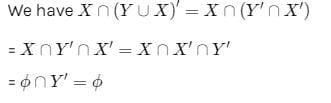CAT  >  Test: Set Theory- 1

# Test: Set Theory- 1

Test Description

## 10 Questions MCQ Test CSAT Preparation for UPSC CSE | Test: Set Theory- 1

Test: Set Theory- 1 for CAT 2022 is part of CSAT Preparation for UPSC CSE preparation. The Test: Set Theory- 1 questions and answers have been prepared according to the CAT exam syllabus.The Test: Set Theory- 1 MCQs are made for CAT 2022 Exam. Find important definitions, questions, notes, meanings, examples, exercises, MCQs and online tests for Test: Set Theory- 1 below.
Solutions of Test: Set Theory- 1 questions in English are available as part of our CSAT Preparation for UPSC CSE for CAT & Test: Set Theory- 1 solutions in Hindi for CSAT Preparation for UPSC CSE course. Download more important topics, notes, lectures and mock test series for CAT Exam by signing up for free. Attempt Test: Set Theory- 1 | 10 questions in 10 minutes | Mock test for CAT preparation | Free important questions MCQ to study CSAT Preparation for UPSC CSE for CAT Exam | Download free PDF with solutions
 1 Crore+ students have signed up on EduRev. Have you?
Test: Set Theory- 1 - Question 1

### Let R be a non-empty relation on a collection of sets defined by ARB if and only if A  ∩ B = Ø Then (pick the TRUE statement)

Detailed Solution for Test: Set Theory- 1 - Question 1

The correct answer is B asLet A={1,2,3
B={4,5}
C={1,6,7}
now
A∩B=∅
B∩C=∅
B∩C=∅ but
A∩C≠∅
R is not transitive.

A∩A=A
R is not reflexive.

A∩B=B∩A
R is symmetric
So,
A is false as
R is not reflexive or transitive
B is true.
C is false because
R is not transitive or reflexive
D is false because
R is symmetric

Test: Set Theory- 1 - Question 2

### The binary relation S =  Φ (empty set) on set A = {1, 2,3}  is

Detailed Solution for Test: Set Theory- 1 - Question 2

Explanation:

• Reflexive : A relation is reflexive if every element of set is paired with itself. Here none of the element of A is paired with themselves, so S is not reflexive.
• Symmetric : This property says that if there is a pair (a, b) in S, then there must be a pair (b, a) in S. Since there is no pair here in S, this is trivially true, so S is symmetric.
• Transitive : This says that if there are pairs (a, b) and (b, c) in S, then there must be pair (a,c) in S. Again, this condition is trivially true, so S is transitive.

Thus, option (D) is correct.

Test: Set Theory- 1 - Question 3

### Which of the following sets are null sets ?

Detailed Solution for Test: Set Theory- 1 - Question 3

There are some sets that do not contain any element at all. For example, the set of months with 32 days. We call a set with no elements the null or empty set. It is represented by the symbol { } or Ø.

Test: Set Theory- 1 - Question 4

Number of subsets of a set of order three is

Detailed Solution for Test: Set Theory- 1 - Question 4

Number of subset = 2n
order 3 = 23
⇒ 8

Test: Set Theory- 1 - Question 5

"n/m" means that n is a factor of m, then the relation T is

Detailed Solution for Test: Set Theory- 1 - Question 5

′/′ is reflexive since every natural number is a factor of itself that in n/n for n∈N.
′/′ is transitive if n is a factor of m and m is a factor of P, then n is surely a factor of P.
However, ′/′ is not symmetric.
example, 2 is a factor of 4 but 4 is not a factor of 2.

Test: Set Theory- 1 - Question 6

The number of elements in the Power set P(S) of the set S = [ [ Φ] , 1, [ 2, 3 ]] is

Test: Set Theory- 1 - Question 7

If A and B are sets and A∪ B= A  ∩ B, then

Test: Set Theory- 1 - Question 8

Let S be an infinite set and S1, S2, S3, ..., Sn be sets such that S1 ∪S2 ∪S3∪ .......Sn = S then

Detailed Solution for Test: Set Theory- 1 - Question 8

Let S = S1 ∪ S2 ∪ S3 ∪ .... Sn .
For S to be infinite set, atleast one of sets Si must be infinite,
if all Si were finite, then S will also be finite.

Test: Set Theory- 1 - Question 9

If X and Y are two sets, then X  ∩ (Y  ∪ X) C equals

Detailed Solution for Test: Set Theory- 1 - Question 9Test: Set Theory- 1 - Question 10

If  f : X -> Y and a, b  ⊆ X, then f (a  ∩ b) is equal to

## CSAT Preparation for UPSC CSE

72 videos|64 docs|92 tests
 Use Code STAYHOME200 and get INR 200 additional OFF Use Coupon Code
Information about Test: Set Theory- 1 Page
In this test you can find the Exam questions for Test: Set Theory- 1 solved & explained in the simplest way possible. Besides giving Questions and answers for Test: Set Theory- 1, EduRev gives you an ample number of Online tests for practice

## CSAT Preparation for UPSC CSE

72 videos|64 docs|92 tests

### How to Prepare for CAT

Read our guide to prepare for CAT which is created by Toppers & the best Teachers